- 10 minutes read

# What a Moving Average Is and How to Compute it in SQL

Want to dive deep into time series data and examine long-term trends? Want to know what a moving average is and how to compute it in SQL? Then this article is for you. I’ll explain a powerful feature in SQL called window functions and show how you can calculate moving averages using them.

## What Is a Moving Average?

The moving average is a time series technique for analyzing and determining trends in data. Sometimes called rolling means, rolling averages, or running averages, they are calculated as the mean of the current and a specified number of immediately preceding values for each point in time. The main idea is to examine how these averages behave over time instead of examining the behavior of the original or raw data points.

Working with moving averages gives us a better representation of the time series, since longer-term trends are much easier to see with moving averages than with raw data points. Since moving averages are often used in financial analysis, I’ll use the data for daily stock prices of a particular company as an example to explain what they are.

Below is the table named `stock_price` that we will use in this article:

dateprice
2020-01-071320
2020-01-081300
2020-01-091300
2020-01-101300
......
2020-06-241086
2020-06-251095
2020-06-261067
2020-06-271067
2020-06-281076
2020-06-291067
2020-06-301067

In this table, we have two columns (`date` and `price`). This is a time series data set, since each stock price is associated with a specific point in time; that is, the price for each day is stored in this table.

The line graph of these prices over time looks like this: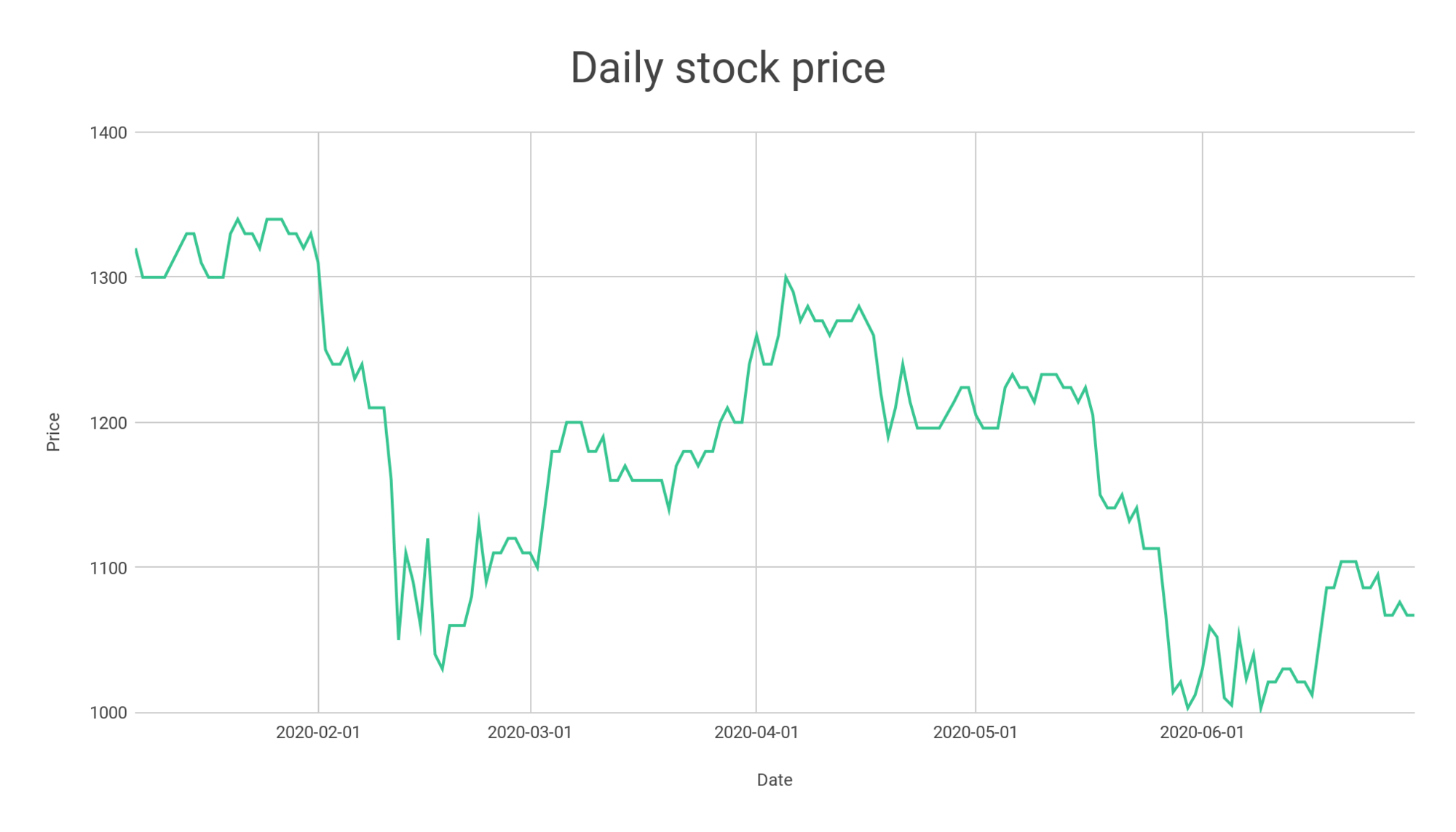We can glean a few things from the table and the graph before we even do any calculations:

• The prices in our table `stock_price` are for the period between January 7, 2020 through June 30, 2020, inclusive.
• The prices range roughly from 1,000 to 1,400 (see the y-axis).
• There are fluctuations in the prices; there are some spikes in February, several spikes in June, etc.

Let’s calculate a moving average for the column `price` and generate a line graph of the averages to see what happens. For this example, we will work with a three-day moving average. To do so, we calculate the average of the stock prices from three consecutive days—the day in question and the two previous days—then repeat the same for each day in the data set. This is a three-day moving average, because we average over a period of three days.

Here is how a three-day moving average is calculated for January 9, 2020: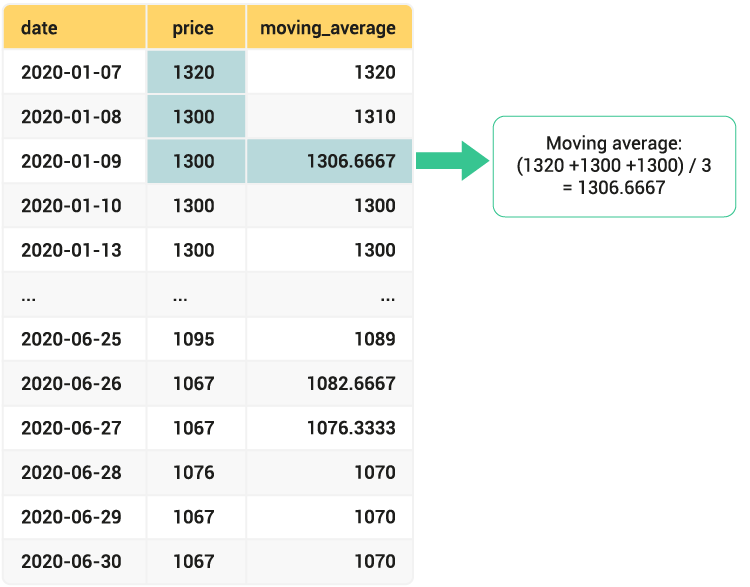For January 9, 2020, the three-day moving average is calculated as the mean of prices from that day (1,300) and the two previous days: January 8 (1,300) and January 7 (1,320). So, the moving average for January 9, 2020 is the average of these three values, or 1,306.66 as shown in the image above.

The moving average is calculated in the same way for each of the remaining dates, totaling the three stock prices from the date in question and the two previous days then dividing that total by 3. For June 30, the three-day moving average is 1,070, the mean of the prices from the dates June 30 (1,067), June 29 (1,067), and June 28 (1,076).

If we plot the original data points and the moving average on a line graph, we get the following: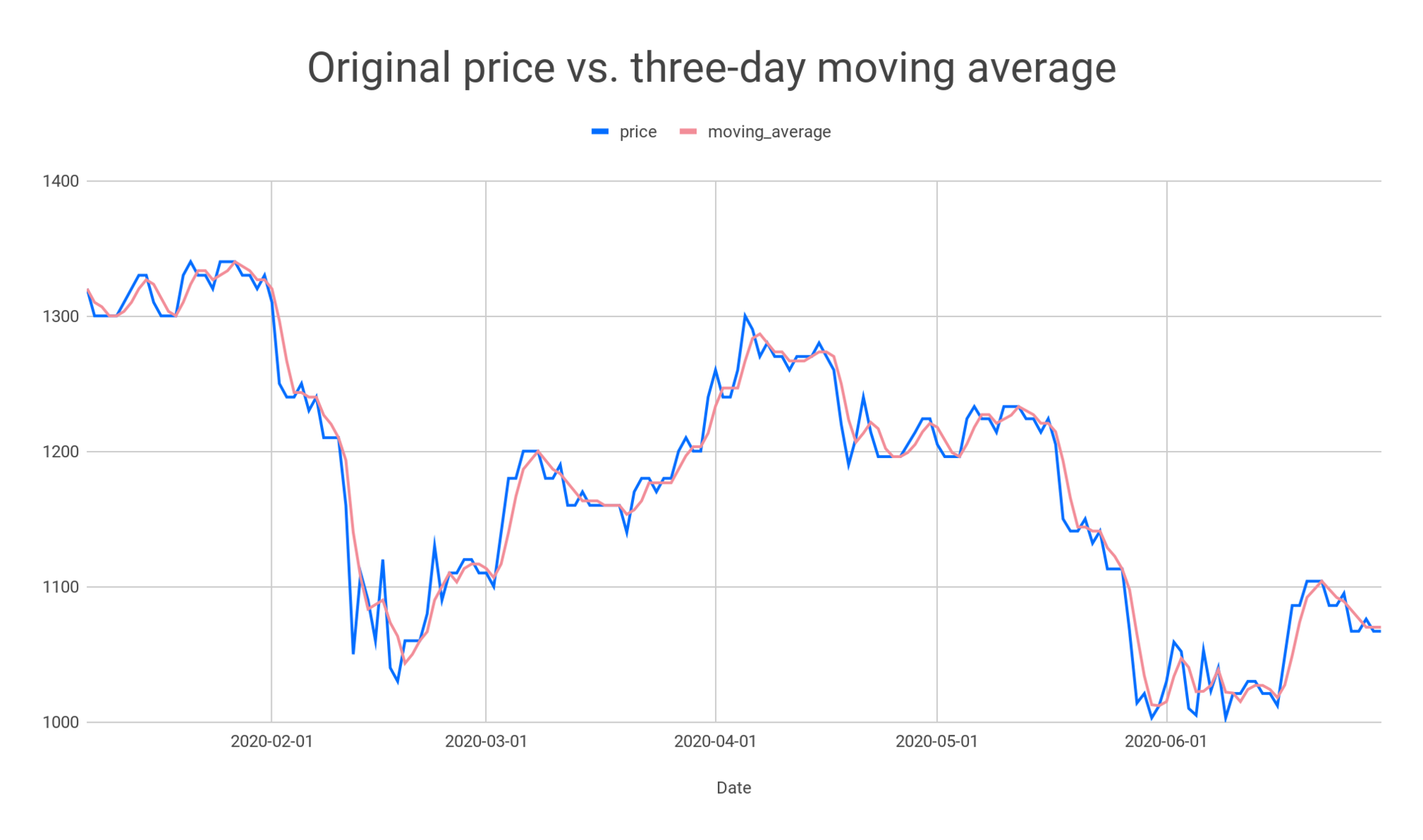The red line represents the moving average, and the blue line represents the original data points. You may notice the red line is smoother and does not have spikes seen in the blue line. This smoothing is the main objective of the moving average technique?—?it is used to remove noise from the data. With less noise, the real trends in time series data are easier to see.

We have calculated the three-day moving average in this example. However, we can calculate it over any period we want, such as the seven-day moving average, the ten-day moving average, and so on.

## Why and Where Moving Averages Are Used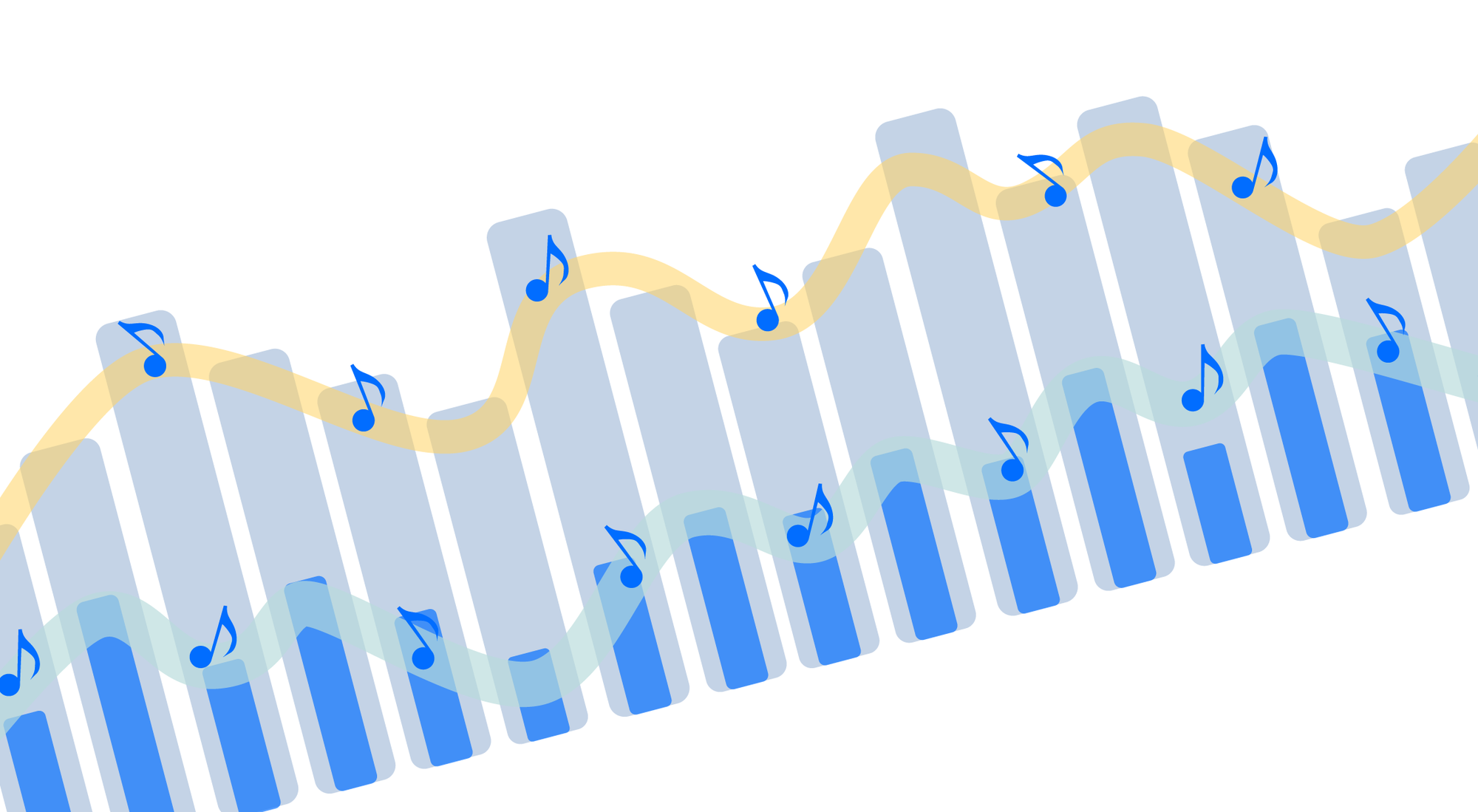Moving averages are widely used in financial and technical trading, such as in stock price analysis, to examine short- and long-term trends. If the stock price stays above the moving average, then we have an uptrend; if it stays below, then traders say that we are in a downtrend. Signals such as uptrends and downtrends inform traders when making decisions about buying or selling stocks.

That said, stock price analysis is not the only use case for the moving average. Other business applications include:

• Sales analysis: Moving averages smooth out fluctuations and spikes in weekly or daily sales.
• Analysis of confirmed COVID-19 cases: Moving averages help show how the number of confirmed cases is changing over time.
• Web traffic analysis: Moving averages help us see long-term trends in number of visits and page views.
• Personal finance: Moving averages help show trends in our spending (for example, looking at the ten-day averages over the last 2 years).

## Moving Averages in SQL

Now that you know what a moving average is, let’s see how to calculate it. In SQL, it is easy with window functions, a special SQL feature that allows you to do aggregations across rows.

While similar to `GROUP BY`, window functions keep all rows when displaying the result; there is no collapsing of rows. Instead, with window functions, we define a frame or a “window” of rows of a given size around the current row then perform some calculation across that window. So, an aggregation is done for each row in a table; each row has its own window over which a calculation is performed.

Below is how the column `moving_average` from our example is calculated in SQL:

```select *,
avg(Price) OVER(ORDER BY Date
ROWS BETWEEN 2 PRECEDING AND CURRENT ROW )
as moving_average
from stock_price;
```

To explain the code in detail:

• We use a window function, denoted with an `OVER` clause. As explained earlier, the rows are not collapsed, and each row has its own window over which a calculation is performed.
• The size of the window in our example is three. For each given row, we take the row itself and the two previous rows, and we calculate the average price from those three rows. This is denoted by the `ROW` keyword in the statement: `ROWS BETWEEN 2 PRECEDING AND CURRENT ROW`. This statement says that, for each row in the table, something is calculated as an aggregation of the current and the previous two rows. This means that the moving average for each row is calculated as the mean price from the given day and the two previous days.
• We have a different window frame for each day. Below, you can see an illustration of the window frame used for the row corresponding to January 9 (in green) and the window frame used for the row corresponding to June 27 (in blue):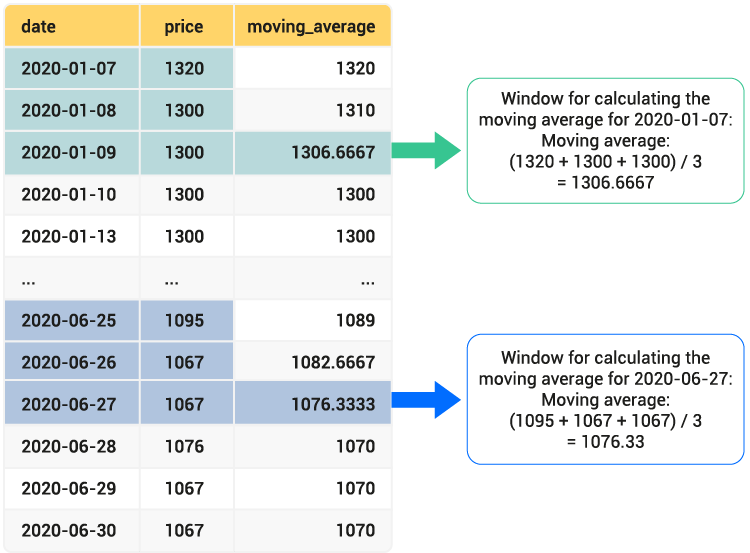• It is important that the data not have any gaps in dates. For each day, we need to calculate the average of the prices from that day and the two previous days. If there are missing dates in the data, this analysis will not make sense.
• The `ORDER BY` keyword inside the `OVER` clause defines the order of the rows over which the moving average should be calculated. In our example, the rows are first sorted by the date column, then the window frame is defined, and the calculation is performed.
• For this example, we do not use the `PARTITION BY` keyword in the `OVER` clause. `PARTITION BY` groups rows into logical chunks by some category, but we are not grouping rows that way here. In effect, our whole data set is just one large partition. Later in this article, we will see an example with a `PARTITION BY`.

So, now you know how to calculate moving averages in SQL! The SQL code above can be used in many other business scenarios; you just need to replace the table and the column names and adjust for the number of rows for which you want to calculate the averages. Everything else can remain the same.

Window functions have a specific syntax, and it takes some time and practice to become familiar with the usage. To learn and practice further, I recommend the window functions course on LearnSQL.com. It is interactive with a lot of exercises, giving you the opportunity to practice and learn the new materials quickly, because you learn by doing! For more information about the course content and window functions themselves, you can read the article SQL Course of the Month: Window Functions. On the same platform, you can also find great articles about when to use window functions and explore some examples.

## Moving Average Over a Specific Number of Days

In the previous example, we calculated a three-day moving average. You can calculate other averages as well, taking any number of previous values you’d like. The higher the number of previous values, the smoother our curve will be. The smaller the number of rows used to calculate the averages, the closer the graph of the moving average will be to that of the original values. There is a huge difference between, for example, two-day and 30-day moving averages.

Before we create a visual to prove this, let’s calculate both of these moving averages:

```select *,
avg(Price) OVER(ORDER BY Date
ROWS BETWEEN 1 PRECEDING AND CURRENT ROW )
as 2day_moving_average,
avg(Price) OVER(ORDER BY Date
ROWS BETWEEN 29 PRECEDING AND CURRENT ROW )
as 30day_moving_average
from stock_price;
```

And now we can visualize how the two lines differ: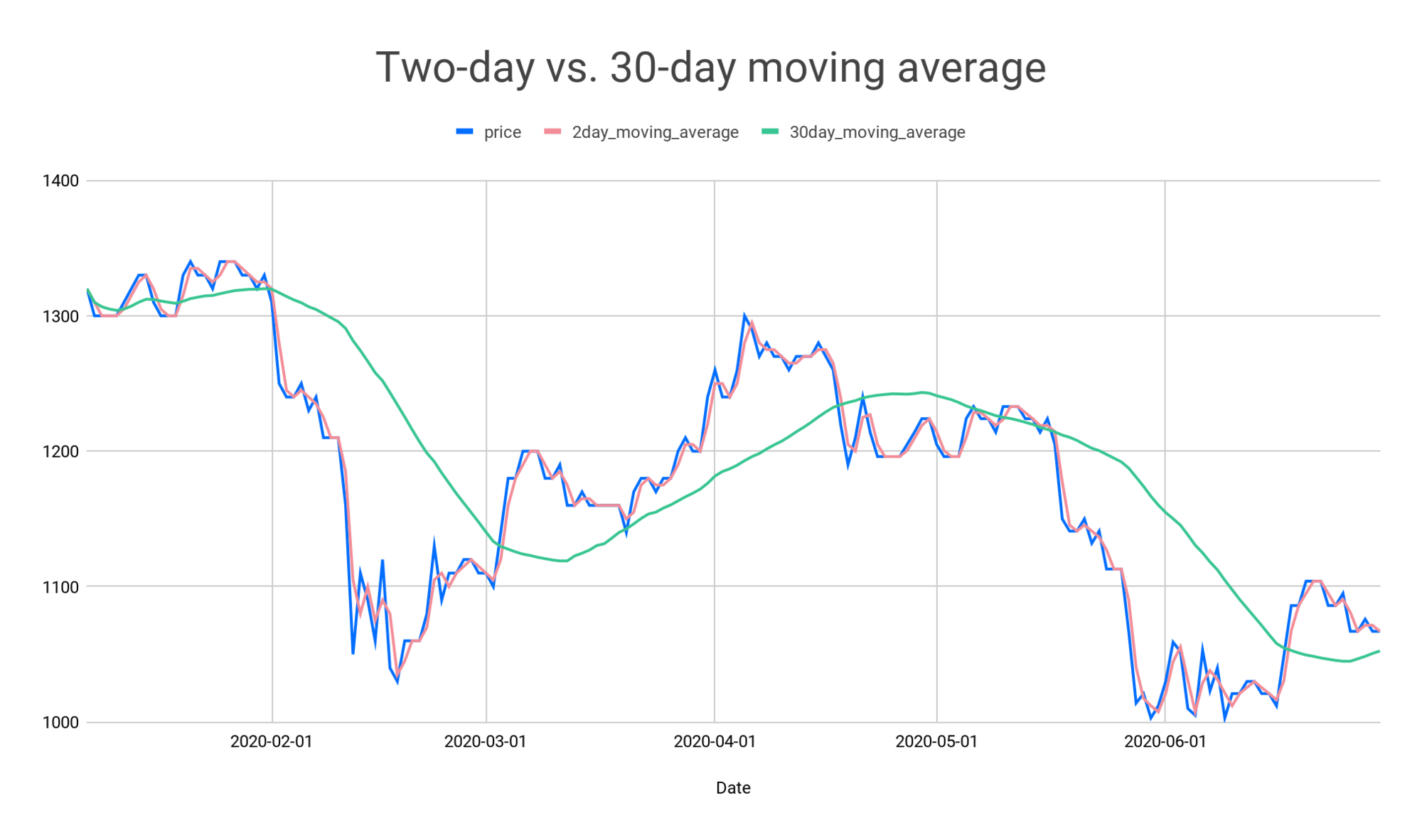The green line represents the 30-day moving average (30 stock prices are used in calculating each average), and the red line represents the two-day average. The red line looks almost like the blue line of the original data points. The green line is smoother and easier to see long-term trends.

Now you may be wondering, how do you know the right number of rows to take? Well, I can’t help you there?—?it really depends on the needs and the situation of the business itself and why you are analyzing the metric in the first place.

## Example: Seven-day Moving Average of COVID Cases

Now let's practice a little more to recap what we have learned so far. COVID-19 is still very real in our lives, so we will calculate the seven-day moving average for the total number of confirmed cases by country. The number of confirmed cases for each day for each country is stored in a table named `confirmed_covid`, in the column `confirmed_day`:

countrydateconfirmed_day
.........
Croatia2020-02-200
Croatia2020-02-210
Croatia2020-02-220
Croatia2020-02-230
Croatia2020-02-240
Croatia2020-02-251
Croatia2020-02-262
Croatia2020-02-270
Croatia2020-02-282
Croatia2020-02-291
.........
Croatia2020-03-120
Croatia2020-03-1313
Croatia2020-03-146
Croatia2020-03-1511
Croatia2020-03-168
.........
Croatia2020-07-18116
Croatia2020-07-1992
Croatia2020-07-2025
Croatia2020-07-2152
Croatia2020-07-22108

To compute the seven-day moving average of the number of confirmed cases, we can do the following:

```SELECT *,
avg(confirmed_day) OVER(
PARTITION BY country
ORDER BY date
ROWS BETWEEN 6 PRECEDING AND CURRENT ROW)
AS 7day_moving_average
FROM confirmed_covid;
```

You may have noticed this code looks just like the one we wrote for the stock price moving average. The one key difference is that we have partitions here?—?each country is a partition, because we are calculating the seven-day averages separately for each country. Otherwise, only the table and the column names are different. You can easily adapt this code to any other business scenario.

Once we run this code and calculate the moving average, we can generate the graph with the line showing the trend for the specific country, from the onset of the pandemic until the month of July. Below is the graph for the country of Croatia: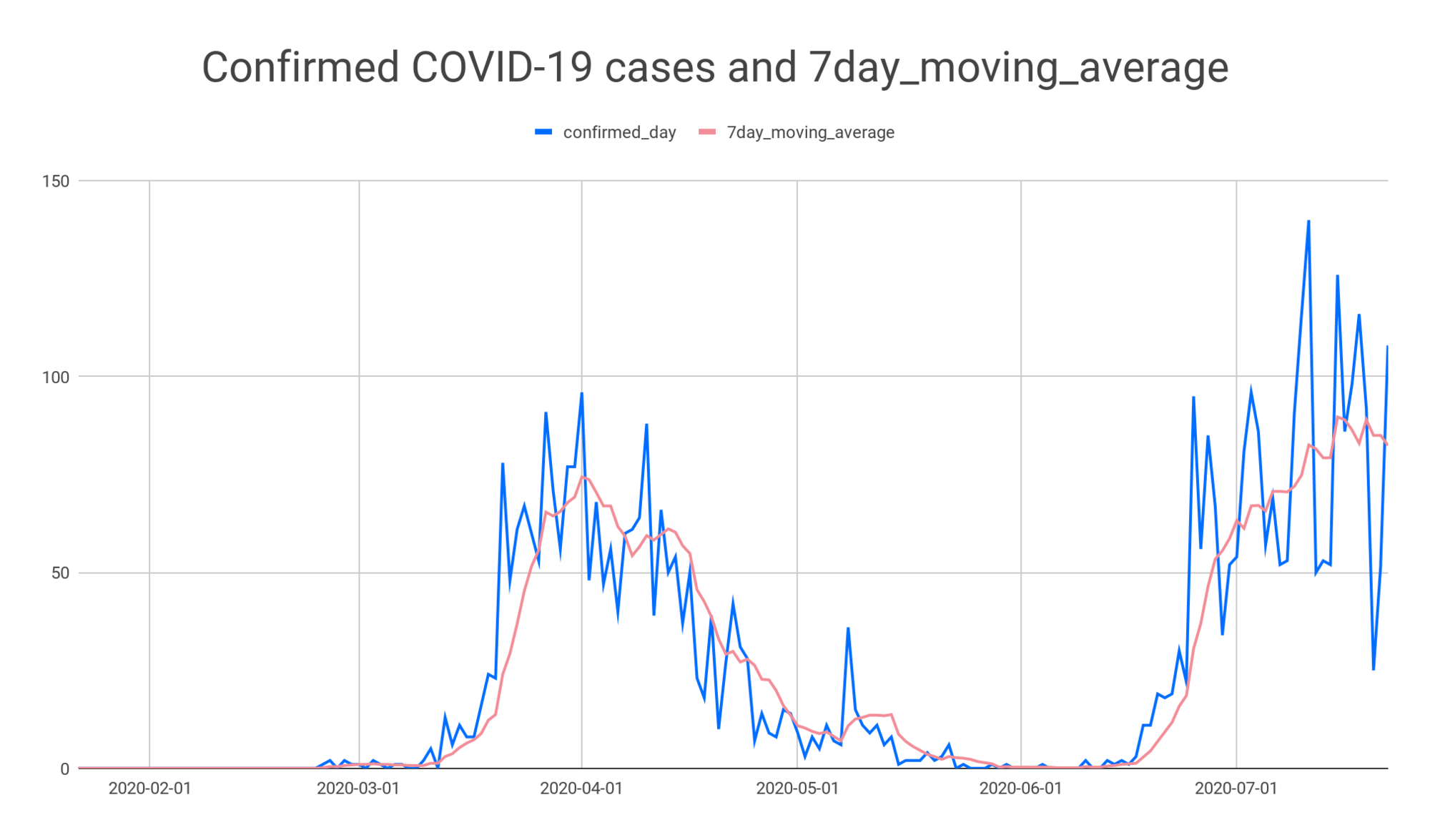Again, we see how the moving average smooths out and reduces spikes and fluctuations compared to the original data points.

You can use other window functions to get powerful insights from the COVID-19 data. If you are interested in more, check out our recent article about how to analyze COVID-19 data using window functions.

## Moving Averages and Window Functions in SQL

In this article, we saw how you can calculate moving averages in SQL using window functions. These averages help us see the real trends better by reducing the amount of noise.

If you would like to practice and learn more about window functions, I recommend the online interactive course on window functions published by LearnSQL.com. This course contains a lot of exercises, so you will definitely put your newly learned skill into practice. And trust me—when it comes to SQL and coding skills, it is important to practice — you learn by doing!